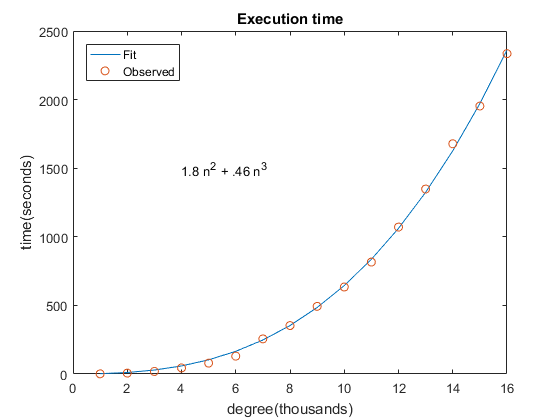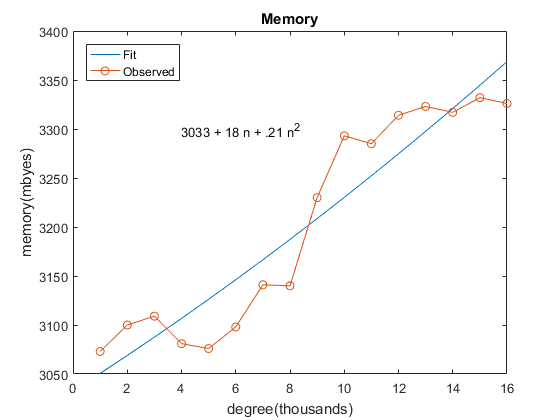# 19 Dubious Ways to Compute the Zeros of a Polynomial

During the SIAM Annual Meeting this summer in Boston there will be a special minisymposium Wednesday afternoon, July 13, honoring Charlie Van Loan, who is retiring at Cornell. (I use "at" because he's not leaving Ithaca.) I will give a talk titled "19 Dubious Way to Compute the Zeros of a Polynomial", following in the footsteps of the paper about the matrix exponential that Charlie and I wrote in 1978 and updated 25 years later. I really don't have 19 ways to compute polynomial zeros, but then I only have a half hour for my talk. Most of the methods have been described previously in this blog. Today's post is mostly about "roots".

### Contents

#### Origin of MATLAB "Roots"

Almost 40 years ago, in the late 1970s, when I was developing the original Fortran-based MATLAB, I wanted to have a command to find the roots of a polynomial. At the time MATLAB was just a primitive matrix calculator. It was not yet a programming environment; I did not yet have M-files or toolboxes.

I had two objectives. I needed to keep the amount of software to a minimum. Mike Jenkins was a friend of mine who had recently submitted his Ph. D. thesis, under Joe Traub's direction, to Stanford, with what is now known as the Jenkins-Traub algorithm for polynomial zero finding. But that would require way too much memory for my MATLAB. And I wanted to turn the tables on the traditional role the characteristic polynomial plays in linear algebra. First year students are taught to compute eigenvalues of a matrix by finding the zeros of the determinant of its companion matrix. Wilkinson had shown that in the presence of roundoff error this is a bad idea. I wanted to emphasize Wilkinson's point by using powerful matrix eigenvalue techniques on the companion matrix to compute polynomial roots.

I already had the EISPACK code for the Francis double shift QR iteration to find the eigenvalues of a Hessenberg matrix. It took just a few more lines to find polynomial roots.

For example, MATLAB represents this polynomial $z^5 - 15 z^4 + 85 z^3 - 225 z^2 + 274 z - 120$ by a vector of coefficients.

   p = [1 -15 85 -225 274 -120]

p =
1   -15    85  -225   274  -120


The roots function finds its roots.

   roots(p)

ans =
5.0000
4.0000
3.0000
2.0000
1.0000


MATLAB does this by forming the companion matrix, which is upper Hessenberg, and computing its eigenvalues.

   A = [15 -85 225 -274 120
eye(4,5)       ]
eig(A)

A =
15   -85   225  -274   120
1     0     0     0     0
0     1     0     0     0
0     0     1     0     0
0     0     0     1     0
ans =
5.0000
4.0000
3.0000
2.0000
1.0000


#### Is "roots" dubious?

In the original documentation for "roots" I wrote

It uses order n^2 storage and order n^3 time. An algorithm designed specifically for polynomial roots might use order n storage and n^2 time. And the roundoff errors introduced correspond to perturbations in the elements of the companion matrix, not the polynomial coefficients.

So, at the time I knew that "roots" had impeccable numerical properties from the matrix eigenvalue point of view, but I wasn't sure about the numerical properties from the polynomial of view. Were we computing the exact roots of some polynomial near the original one? Maybe not. It depends crucially upon the scaling.

"Roots" is possibly dubious numerically, and certainly dubious from a computation cost point of view.

#### Balancing

Almost twenty years later, in 1994 and 1995, a pair of papers, one by Nick Trefethen and K. C. Toh, and one by Alan Edelman and H. Murakami, finally gave "roots" a passing grade numerically, provided the companion matrix is first scaled by balancing.

Balancing was introduced by Parlett and Reinsch in the Algol procedures that spawned EISPACK. Balancing is a diagonal similarity transformation by a matrix of powers of two to attempt to bring a nonsymmetric matrix closer to symmetric by making its row and column norms equal. It is available in MATLAB through the function balance and is used by default by eig.

To see the importance of balancing, consider the companion matrix of $W_{20}$, the Wilkinson polynomial of degree 20 whose roots are the integers from 1 to 20. The coefficients of this polynomial are huge. The constant term is 20!, which is 2432902008176640000. But that's not the largest. The coefficient of $z^2$ is 13803759753640704000. Five of the coefficients, those of $z^3$ through $z^7$ are so large that they cannot be exactly represented as double precision floating pointing numbers. So

roots(poly(1:20))


starts with a companion matrix that has coefficients of magnitude 10^19 along its first row and ones on its subdiagonal. It is seriously nonsymmetric.

The elements of the balanced matrix are much more reasonable. They range from just 1/4 to 256. This is the matrix involved in the actual eigenvalue calculation. All the scaling action is captured in the diagonal similarity, whose elements range from $2^{-35}$ to $2^{27}$. This matrix is only involved in scaling the eigenvectors.

So I was lucky on the numerical side. Balancing turned out to provide "roots" satisfactory stability.

#### Execution time

Let's measure the execution time and memory requirements for "roots" with polynomials of very large degree -- tens of thousands. Here I just wanted to see how bad it is. Short answer: it takes "roots" almost 40 minutes to find the zeros of a polynomial of order sixteen thousand on my Lenovo X250 laptop.

Here is the experiment. Polynomials of degree 1000*n for n = 1:16.

T = zeros(16,1);
for n = 1:16
n
r = randn(1,1000*n);
tic
roots(r);
t = toc
T(n) = t;
!systeminfo | grep 'In Use'
end


As expected, the observed exection times are fit nicely by a cubic in the polynomial degree.

   time_roots

c =
0
1.8134
0.4632#### Memory requirements

On the other hand, the measured virtual memory requirements are all other the map. A least squares fit by a quadratic in the polynomial degree $n$ shows very little dependence on the $n^2$ term. It's fair to say that on today's machines memory requirements are not a significant restriction for this algorithm.

   memory_roots

c =
1.0e+03 *
3.0328
0.0177
0.0002#### AMVW

AMVW are the initials of the last names of Jared Aurentz, Thomas Mach, Raf Vandebril, and David Watkins. It is also the name of their Fortran code for what they claim is a competitor to "roots". Their recent paper in SIMAX, referenced below, summarizes all the work that has been done on computing the eigenvalues of companion matrices, exploiting their special structure.

They also present their own algorithm where the companion matrix is never formed explicitly, but is represented as a product of Givens rotations. The QR algorithm is carried out on this representation. As a result only $O(n)$ storage and $O(n^2)$ operations are required.

But their software is in Fortran. I was a big Fortran fan for a long time. I used to be considered one of the Fortran gurus at MathWorks. I now don't even have access to a Fortran compiler. So I can't try their stuff myself.

#### Fiedler Companion Matrix

So far I've been talking about the classic companion matrix where the polynomial coefficients occupy the entire first or last row or column. In 2003 Miroslav Fiedler introduced another version of a companion matrix, a pentadiagonal matrix with the polynomial coefficients alternating with zeros on the super- and subdiagonal and ones and zeros alternating on the next diagonals of the nonsymmetric pentadiagonal matrix. I wrote about it my blog just before last Christmas.

Here's an example, W_8. First, the coefficients.

   c = poly(1:8)'

c =
1
-36
546
-4536
22449
-67284
118124
-109584
40320


The Fiedler companion matrix.

   F = fiedler(c(2:end))

F =
Columns 1 through 6
36        -546           1           0           0           0
1           0           0           0           0           0
0        4536           0      -22449           1           0
0           1           0           0           0           0
0           0           0       67284           0     -118124
0           0           0           1           0           0
0           0           0           0           0      109584
0           0           0           0           0           1
Columns 7 through 8
0           0
0           0
0           0
0           0
1           0
0           0
0      -40320
0           0


Balance it.

   B = balance(F)

B =
Columns 1 through 7
36.0000  -17.0625   16.0000         0         0         0         0
32.0000         0         0         0         0         0         0
0    8.8594         0   -5.4807    8.0000         0         0
0    8.0000         0         0         0         0         0
0         0         0    2.0533         0   -0.9012    1.0000
0         0         0    4.0000         0         0         0
0         0         0         0         0    0.8361         0
0         0         0         0         0    0.5000         0
Column 8
0
0
0
0
0
0
-0.6152
0


Check the eigenvalues

   e = eig(B)

e =
8.0000
7.0000
6.0000
5.0000
4.0000
3.0000
2.0000
1.0000


If we could find a way to compute the eigenvalues and eigenvectors of the Fiedler companion matrix while exploiting its structure, then we would have a gorgeous algorithm. Beresford Parlett tells me that he's working on it.

#### References

Kim-Chuan Toh and Lloyd N. Trefethen, "Pseudozeros of polynomials and pseudospectra of companion matrices," Numer. Math., 68, 1994.

Alan Edelman and H. Murakami, "Polynomial roots from companion matrices," Mathematics of Computation, 64, 1995. <http://www.ams.org/journals/mcom/1995-64-210/S0025-5718-1995-1262279-2/>

Jared L. Aurentz, Thomas Mach, Raf Vandebril, and David S. Watkins, "Fast and backward stable computation of roots of polynomials," SIAM J. Matrix Anal. Appl., 36, 2015. <http://www.math.wsu.edu/faculty/watkins/pdfiles/AMVW15_SIMAX.pdf>

Miroslav Fiedler, A note on companion matrices, Linear Algebra and its Applications 372 (2003), 325-331.

Published with MATLAB® R2016a

|

### 댓글

댓글을 남기려면 링크 를 클릭하여 MathWorks 계정에 로그인하거나 계정을 새로 만드십시오.